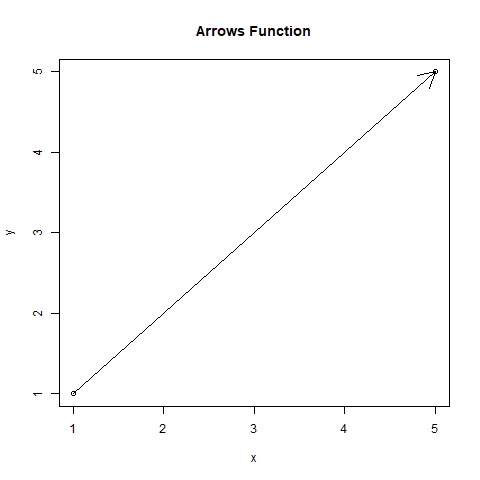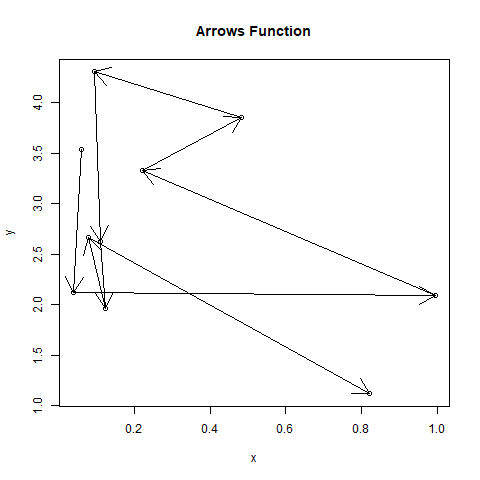GFG App
Open AppBrowser
Continue

# Plot Arrows Between Points in a Graph in R Programming – arrows() Function

`arrows()` function in R Language is used to create arrows between the points on the graph specified.

Syntax: arrows(x0, y0, x1, y1, length)

Parameters:
x0: represents x-coordinate of point from which to draw the arrow
y0: represents y-coordinate of point from which to draw the arrow
x1: represents x-coordinate of point to which the arrow is drawn
y1: represents y-coordinate of point to which the arrow is drawn
length: represents length of the edge of the arrow head (in inches)

Example 1:

 `# Specifying points ` `x0 <- 1 ` `y0 <- 1 ` `x1 <- 5 ` `y1 <- 5 ` `x <- ``c``(x0, x1) ` `y <- ``c``(y0, y1) ` ` `  `# Output to be present as PNG file ` `png``(file = ``"arrows1GFG.png"``) ` ` `  `# Create plot graph ` `plot``(x, y, main = ``"Arrows Function"``) ` ` `  `# Create arrow between the points ` `arrows``(x0, y0, x1, y1) ` ` `  `# Saving the file ` `dev.off``() `

Output:Example 2:

 `# Specifying points ` `x <- ``runif``(10, 0, 1) ` `y <- ``runif``(10, 1, 5) ` ` `  `# Output to be present as PNG file ` `png``(file = ``"arrows2GFG.png"``) ` ` `  `# Create plot graph ` `plot``(x, y, main = ``"Arrows Function"``) ` ` `  `# Create arrow between the points ` `s <- ``seq``(``length``(x) - 1) ` `arrows``(x[s], y[s], x[s + 1], y[s + 1]) ` ` `  `# Saving the file ` `dev.off``() `

Output:My Personal Notes arrow_drop_up